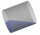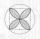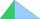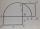# Cylinder horizontally

The cylinder with a diameter of 3 m and a height/length of 15 m is laid horizontally. Water is poured into it, reaching a height of 60 cm below the axis of the cylinder. How many hectoliters of water is in the cylinder?

Result

V =  267.527 hl

#### Solution:Leave us a comment of example and its solution (i.e. if it is still somewhat unclear...):Be the first to comment!#### To solve this example are needed these knowledge from mathematics:

See also our right triangle calculator. Do you want to convert length units? Do you know the volume and unit volume, and want to convert volume units? See also our trigonometric triangle calculator.

## Next similar examples:

1. CylinderCalculate the dimensions of rotating cylindrical container with volume 2 l, if height of container is equal to the diameter of the base.
2. Horizontal Cylindrical SegmentHow much fuel is in the tank of horizontal cylindrical segment with a length 10m, width of level 1 meter and level is 0.2 meters below the upper side of the tank?
3. JarFrom the cylinder shaped jar after tilting spilled water so that the bottom of the jar reaches the water level accurately into half of the base. Height of jar h = 7 cm and a jar diameter D is 12 cm. How to calculate how much water remains in the jar?
4. The potDiameter of the pot 38 cm. The height is 30 cm. How many liters of water can fit in the pot?
5. Cylinder surface, volumeThe area of the cylinder surface and the cylinder jacket are in the ratio 3: 5. The height of the cylinder is 5 cm shorter than the radius of the base. Calculate surface area and volume of cylinder.
6. Conserving waterCalculate how many euros are spent annually on unnecessary domestic hot water, which cools during the night in pipeline. Residential house has 129 m of hot water pipelines 5/8" and the hot water has a price of 7 Eur/m3.
7. Circular poolThe 3.6-meter pool has a depth of 90 cm. How many liters of water is in the pool?
8. Cylindrical tank 2If a cylindrical tank with volume is used 12320cm raised to the power of 3 and base 28cm is used to store water. How many liters of water can it hold?
9. Juice boxThe juice box has a volume of 200ml with its base is an isosceles triangle with sides a = 4,5cm and a height of 3,4cm. How tall is the box?
10. QuatrefoilCalculate area of the quatrefoil which is inscribed in a square with side 6 cm.
11. Right angledFrom the right triangle with legs 12 cm and 20 cm we built a square with the same content as the triangle. How long will be side of the square?
12. Circle arcCalculate the area of the circular arc in m2 where the diameter is 290 dm and a central angle is 135°. Result round to three decimal places.
13. Theorem proveWe want to prove the sentence: If the natural number n is divisible by six, then n is divisible by three. From what assumption we started?
14. Chord - TS v2The radius of circle k measures 87 cm. Chord GH = 22 cm. What is TS?
15. Chord - TSThe radius of circle k measures 68 cm. Arc GH = 47 cm. What is TS?
16. Same areaThere is a given triangle. Construct a square of the same area.
17. TriangleCalculate the area of ​​the triangle ABC if b = c = 17 cm, R = 19 cm (R is the circumradius).